# 2015年数模A题太阳影子定位学习笔记

To be continue … …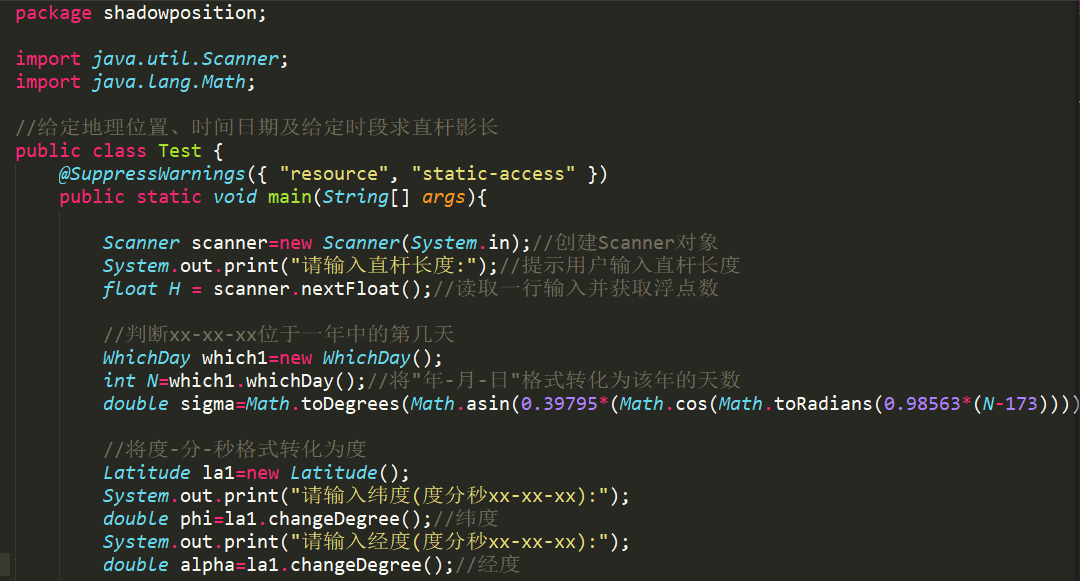## 四、模型假设

1. 假设海拔对影子长度的影响可忽略;
2. 问题四中相机拍摄的角度对影子实际长度的测量影响不大;
3. 题中所给的直杆顶点坐标数据是实时实地测量得的，是真实可靠的;
4. 忽略大气折射的影响;
5. 地球自转为匀角速度;
6. 太阳光线为平行光.

## 五、定义与符号说明

### 5.1 符号说明

h h 太阳高度角
L L 直杆长度
d d 影子长度
φ \varphi 所测地点的地理纬度
α \alpha 所测地点的地理经度
δ \delta 赤纬角
ω \omega 时角
t ′ t' 所测地点时间
t t 北京时间
N日数(以1月1日为第一天，依次类推)

## 六、模型的建立与求解

### 6.1 模型一：基于太阳赤纬与时差计算的结构方程模型——问题一的求解

#### 6.1.1 建模准备——影子长度计算公式

tan ⁡ h = L d (6.1) \tan h=\frac{L}{d}\tag{6.1}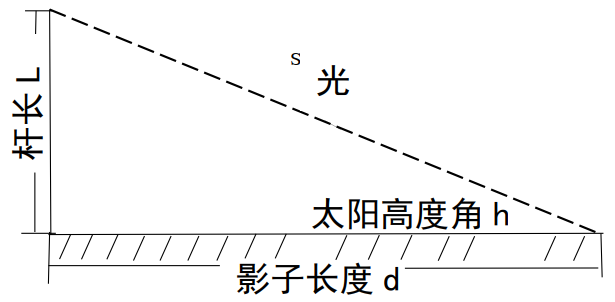sin ⁡ h = sin ⁡ φ sin ⁡ δ + cos ⁡ φ cos ⁡ δ cos ⁡ ω (6.2) \sin h=\sin \varphi \sin\delta +\cos \varphi \cos \delta \cos \omega\tag{6.2} 赤纬角计算公式
sin ⁡ δ = 0.39795 ⋅ cos ⁡ [ 0.9853 ⋅ ( N − 173 ) ] (6.3) \sin \delta=0.39795\cdot \cos[0.9853\cdot (N-173)]\tag{6.3}
δ = − 23.4 4 ∘ ⋅ cos ⁡ ( 360 365 ⋅ ( N + 10 ) ) \delta=-23.44^{\circ}\cdot \cos\big (\frac{360}{365}\cdot(N+10)\big)\\ 时角是以正午12点为0°开始算，且时角每一小时增加15°，即
ω = ( t ′ − 12 ) ⋅ 1 5 ∘ (6.4) \omega=(t'-12)\cdot15^{\circ}\tag{6.4} 在不同纬度时间不同，计算所测地点时间利用北京时间转化。经度相差1°，时差相差1小时，可得所测地点时间计算公式如下
t ′ = t − 8 + α 1 5 ∘ (6.5) t'=t-8+\frac{\alpha}{15^{\circ}}\tag{6.5} 联立式(6.1)～(6.5)，得到影子长度的计算公式
{ d = L ⋅ cot ⁡ [ arcsin ⁡ ( sin ⁡ φ sin ⁡ δ + cos ⁡ φ cos ⁡ δ cos ⁡ ω ) ] δ = − 23.4 4 ∘ ⋅ cos ⁡ ( 360 365 ⋅ ( N + 10 ) ) ω = ( t ′ − 12 ) ⋅ 1 5 ∘ t ′ = t − 8 + α 1 5 ∘ (6.6) \begin{aligned} \begin{cases} d=L\cdot\cot\big[\arcsin(\sin\varphi\sin\delta+\cos\varphi\cos\delta\cos\omega)\big]\\ \delta=-23.44^{\circ}\cdot\cos(\frac{360}{365}\cdot(N+10))\\ \omega=(t'-12)\cdot15^{\circ}\\ t'=t-8+\frac{\alpha}{15^{\circ}} \end{cases} \end{aligned}\tag{6.6}

#### 6.1.2 影子长度关于各个参数变化规律

##### (1) 纬度 φ \varphi 与日数 N N 一定，影子长度 d d 随时间 t ′ t' 变化规律

t ′ = t − 8 + 116 + 23 / 60 + 29 / 3600 1 5 ∘ (6.7) t'=t-8+\frac{116+23/60+29/3600}{15^{\circ}}\tag{6.7} 式中， t t 为北京时间。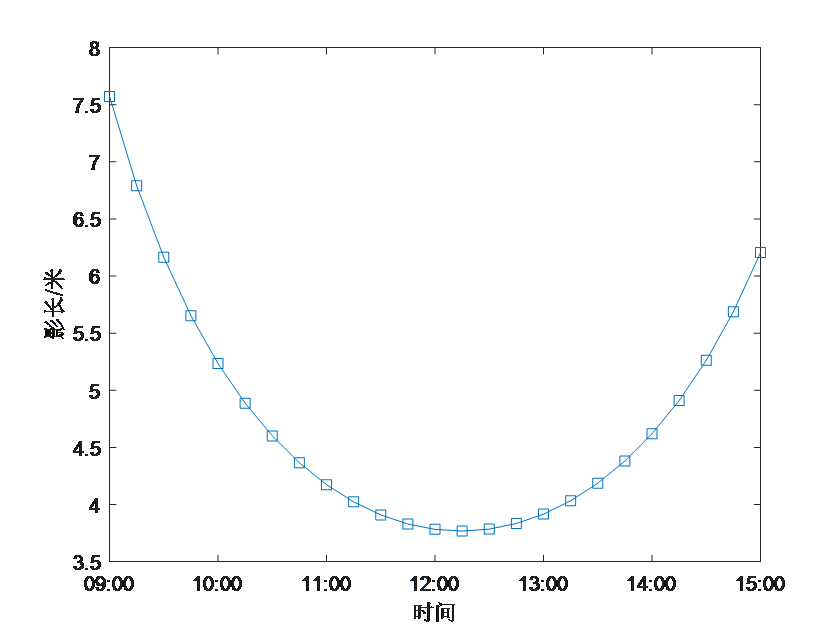clear
clc
%问题一
H=3;%杆长3m
%计算2015年10月22日北京赤纬-11.5637度
sigma=asind(0.39795*cosd(0.98563*(295-173)));
phi=dms2degrees([39,54,26]);%纬度:度分秒转化为度
alpha=dms2degrees([116,23,29]);%经度:度分秒转化为度
t=9:0.25:15;%每间隔15min测量一次
omega=15*t+alpha-300;
L=H*cotd(asind(sind(phi)*sind(sigma)+cosd(phi)*cosd(sigma).*cosd(omega)));
num=length(L); %数据的点数是数列的长度
N0=datenum([0 0 0 9 0 0]); %起始时间9:00
dN=datenum([0 0 0 0 15 0]);
N=N0+(0:num-1)*dN; %根据数据点数，产生15分钟间隔时间点
plot(N,L,'s-');
datetick(gca,'x','HH:MM');
xlabel("时间")
ylabel("影长/米")

##### (2) 纬度 φ \varphi 与时间 t ′ t' 一定，影子长度 d d 随天数N的变化规律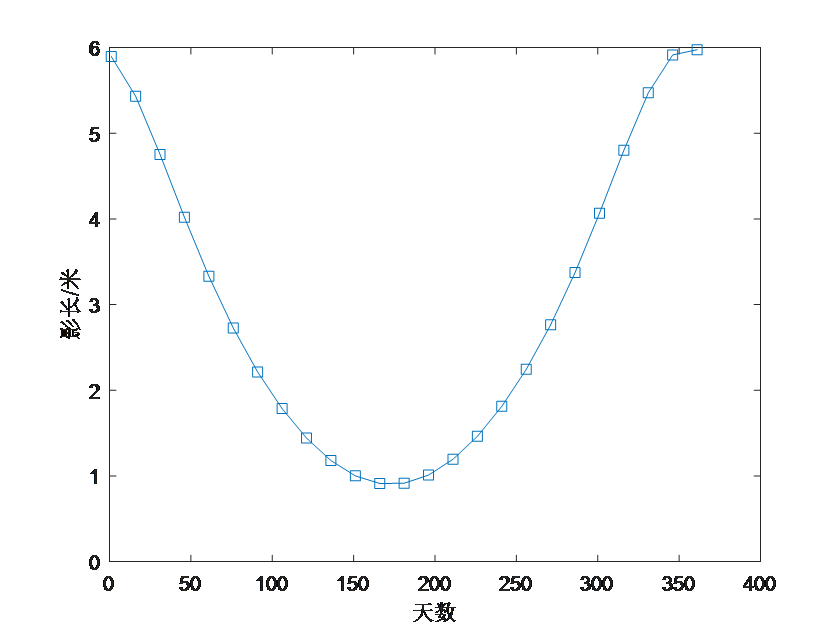clear
clc
%问题一第2小问灵敏度分析2,此时改变天数N
H=3;%杆长3m
%计算随天数N改变时北京的赤纬
N=1:15:365;
sigma=asind(0.39795.*cosd(0.98563.*(N-173)));
phi=dms2degrees([39,54,26]);%纬度:度分秒转化为度
alpha=dms2degrees([116,23,29]);%经度:度分秒转化为度
t=12;%以正午12点为例进行灵敏度分析
omega=15*t+alpha-300;
L=H*cotd(asind(sind(phi).*sind(sigma)+cosd(phi).*cosd(sigma)*cosd(omega)));
m=min(L);
d=find(L==min(L));
plot(N,L,'s-');
xlabel("天数")
ylabel("影长/米")

##### (3) 日数 N N 与时间 t ′ t' 一定，影子长度 d d 随天数 N N 变化规律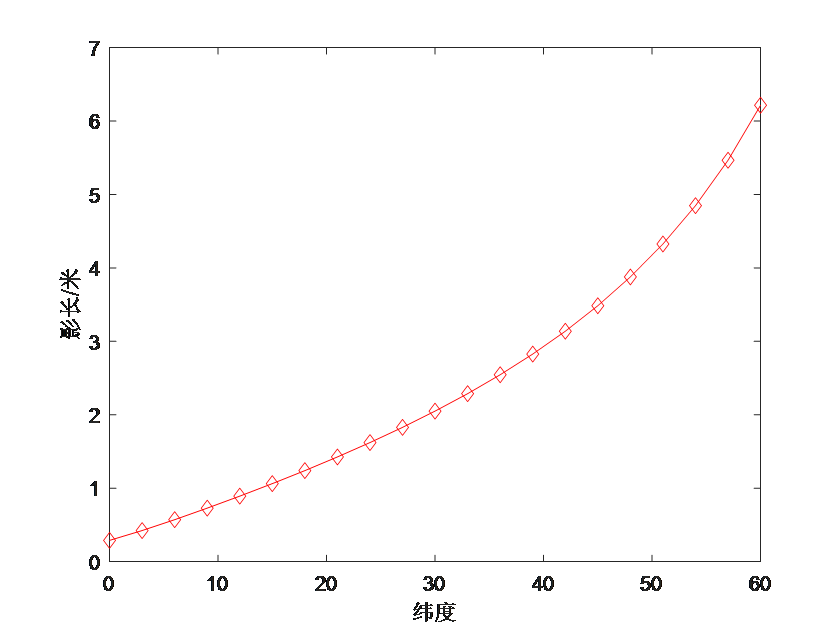clear
clc
%问题一第3小问灵敏度分析3,此时改变维度phi
H=3;%杆长3m
%计算随维度phi改变时北京的赤纬
N=275;
sigma=asind(0.39795*cosd(0.98563.*(N-173)));
phi=[0:3:60];%纬度
%phi=dms2degrees([phi,0,0]);
alpha=dms2degrees([116,23,29]);%经度:度分秒转化为度
t=12;%以正午12点为例进行灵敏度分析
omega=15*t+alpha-300;
L=H*cotd(asind(sind(phi)*sind(sigma)+cosd(phi)*cosd(sigma)*cosd(omega)));
plot(phi,L,'rd-');
xlabel("纬度")
ylabel("影长/米")


### 6.2 模型二：基与最小二乘法模型——问题二求解

#### 6.2.1 建模准备

a r c c o t ( L / d ) = h (6.8) arccot(L/d)=h \tag{6.8} 两边用 sin ⁡ \sin 函数作用，得
sin ⁡ ( a r c c o t ( L / d ) ) = sin ⁡ φ sin ⁡ δ + cos ⁡ φ cos ⁡ δ cos ⁡ ω (6.9) \sin(arccot(L/d) ) =\sin\varphi\sin\delta+\cos\varphi\cos\delta\cos\omega \tag{6.9} 整理得
L = d ⋅ [ 1 ( sin ⁡ φ sin ⁡ δ + cos ⁡ φ cos ⁡ δ cos ⁡ ω ) 2 ] 1 / 2 (6.10) L=d\cdot [\frac{1}{(\sin\varphi\sin\delta+\cos\varphi\cos\delta\cos\omega)^{2}}]^{1/2}\tag{6.10} 式(5.10)中含有 3 个未知数 H H φ \varphi ω \omega ，通过方程组求解未知数， 过程繁琐复杂。 因此，引入最小二乘法的曲线拟合方法。给定平面上的点 ( x i , y i ) (x_{i},y_{i}) i = 1 , 2 , ⋯   , n i=1,2,\cdots,n ，用最小二乘法求 f ( x ) f(x) ，使 δ = ∑ δ i 2 \delta=\sum\delta_{i}^{2} 达到最小。其中 δ i \delta_{i} 为点 ( x i , y i ) (x_{i},y_{i}) 与曲线 y = f ( x ) y=f(x) 的距离。曲线拟合的实际含义是寻求一个函数 y = f ( x ) y=f(x) ，使在某种准则下与所有数据点最为接近，即曲线拟合得最好。最小二乘准则就是使所有散点到曲线的距离平方和最小，拟合时选用一些简单的“基函数”（例如幂函数，三角函数等等） φ 0 ( x ) \varphi_{0}(x) φ 1 ( x ) \varphi_{1}(x) ⋯ \cdots φ m ( x ) \varphi_{m}(x) 来线性表示
f ( x ) = c 0 φ 0 + c 1 φ 1 + ⋯ + c m φ m (6.11) f(x)=c_{0}\varphi_{0}+c_{1}\varphi_{1}+\cdots+c_{m}\varphi_{m}\tag{6.11} 式(6.10)为依据太阳影子长度 d d 含杆长 L L 、纬度 φ \varphi 的理论公式， 建立的最小二乘法模型。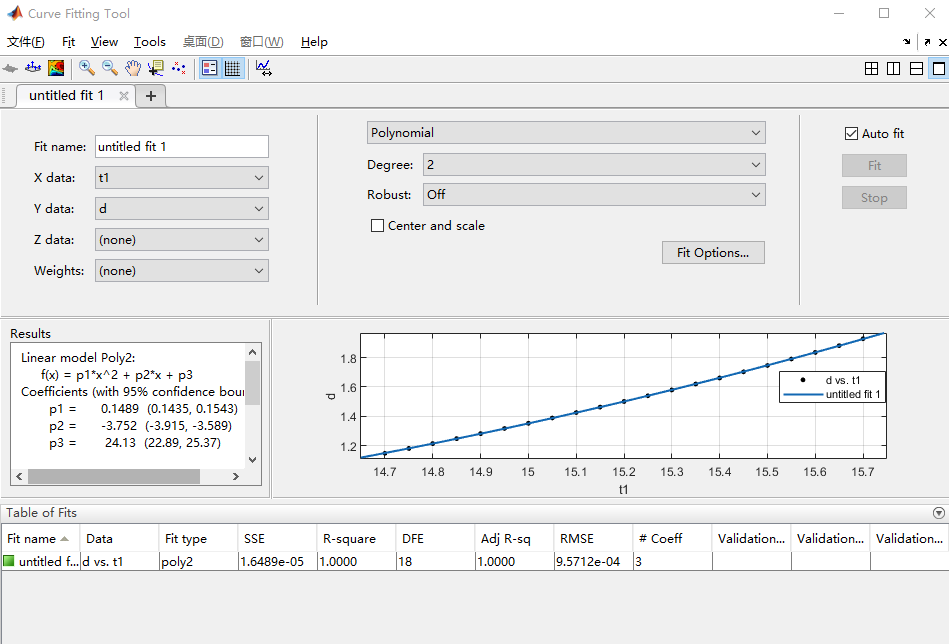#### 6.2.2 数据的预处理

14.71.149615.051.389415.41.6613
14.751.182215.11.426215.451.7033
14.81.213515.151.464515.51.7462
14.851.249115.21.501515.551.7901
14.91.283215.251.540215.61.8350
14.951.318015.31.579915.651.8809
15.01.353415.351.620115.71.9279

y = 0.1489 t 2 − 3.752 t + 24.13 (6.12) y=0.1489t^{2}-3.752t+24.13\tag{6.12} 式 (6.12) 中 ， 拟 合 精 度 指 标 SSE 为 1.669 × 1 0 − 5 1.669\times10^{-5} ， R-square 为1，Adjuster-square 为1， RMSE 为 9.63 × 1 0 − 4 9.63\times10^{-4} 。SSE 及 RMSE 越接近0， R-square和 Adjuster-square 越接近1， 表明拟合效果越好。 式(5.12)为抛物线， 较好地反映出物体影子长度随时角变化的趋势， 并用 MATLAB 绘出式(6.12)函数曲线， 如图 6 所示。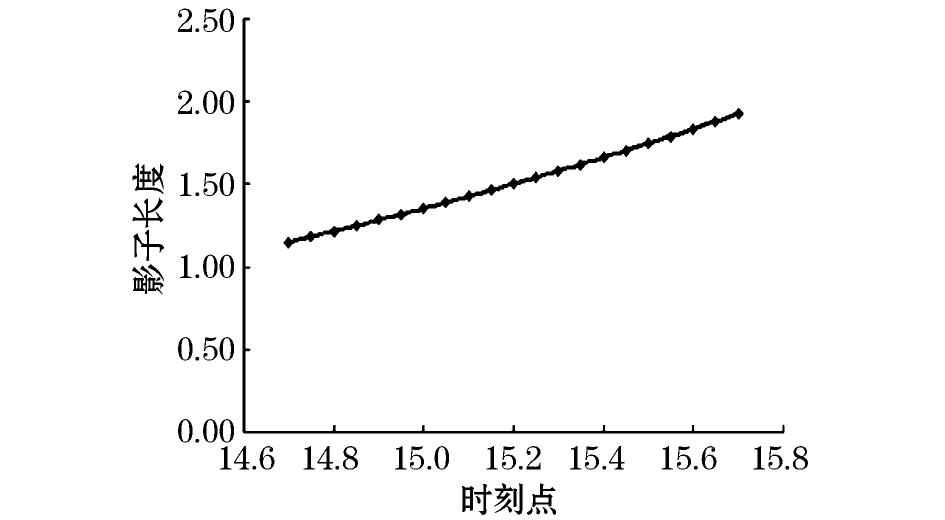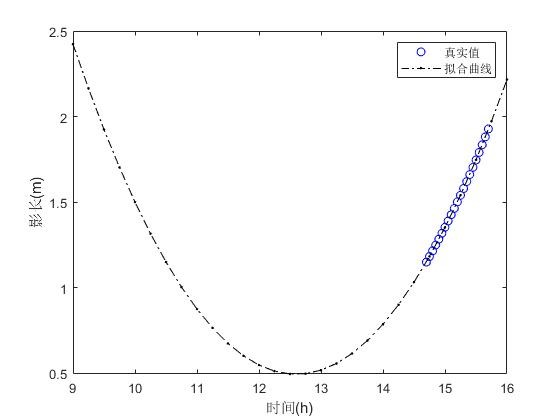clear
clc
x=[1.0365,1.0699,1.1038,1.1383,1.1732,1.2087,1.2448,...
1.2815,1.3189,1.3568,1.3955,1.4349,1.4751,1.516,...
1.5577,1.6003,1.6438,1.6882,1.7337,1.7801,1.8277
];%附件1的x坐标
y=[0.4973,0.5029,0.5085,0.5142,0.5198,0.5255,0.5311,...
0.5368,0.5426,0.5483,0.5541,0.5598,0.5657,0.5715,...
0.5774,0.5833,0.5892,0.5952,0.6013,0.6074,0.6135
];%附件1的y坐标
d=sqrt(x.^2+y.^2);%附件1的影长
t1=14.7:0.05:15.7;
plot(t1,d,'bo');hold on;
t=9:0.25:16;
y=0.1489*t.^2-3.752*t+24.13;
plot(t,y,'k.-.');
xlabel("时间(h)");ylabel("影长(m)");
legend("真实值","拟合曲线");


#### 6.2.3 问题二的求解——基于半搜索算法

##### 2. 模型阶段二：半搜索算法思路

clear
clc
%第二问方法一
L=1:0.01:3;%设置杆长的搜索范围
phi=-20:0.01:30;%设置搜索的纬度范围
N=31+28+31+18;%计算该日期下的日数
delta=asind(0.39795*cosd(0.98563*(N-173)));%该日下的赤纬角
A=sind(delta);B=cosd(delta);
d=0.4934;K={};k=0;%当地正午12点对应的杆影长度
for i=1:length(L)
for  j=1:length(phi)
D=L(i)*cotd(asind(A*sind(phi(j))+B*cosd(phi(j))));
if abs(d-D)<0.000008
k=k+1;
list=[L(i),phi(j)];
K{k}=list;list=[];
else
continue;
end
end
end

##### 3. 方法二：循环遍历搜索法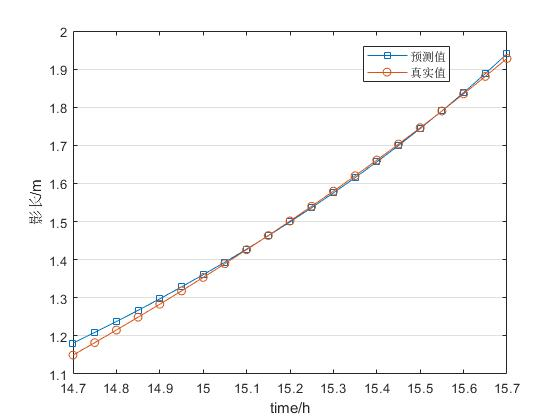clear
clc
%第2问方法二遍历搜索
x=[1.0365,1.0699,1.1038,1.1383,1.1732,1.2087,1.2448,...
1.2815,1.3189,1.3568,1.3955,1.4349,1.4751,1.516,...
1.5577,1.6003,1.6438,1.6882,1.7337,1.7801,1.8277];%杆影的x坐标
y=[0.4973,0.5029,0.5085,0.5142,0.5198,0.5255,0.5311,...
0.5368,0.5426,0.5483,0.5541,0.5598,0.5657,0.5715,...
0.5774,0.5833,0.5892,0.5952,0.6013,0.6074,0.6135];%杆影的y坐标
d=sqrt(x.^2+y.^2);%杆影长
K={};k=0;%KK=[];KKK=[];
alpha=0:1:180;%经度
phi=-90:1:90;%纬度
L=1:0.1:3;%杆长
N=31+28+31+18;%计算该日期下的日数
delta=asind(0.39795*cosd(0.98563*(N-173)));%该日下的赤纬角
t=14.7:0.05:15.7;%拍摄时的北京时间
A=sind(delta);B=cosd(delta);
for i=1:length(alpha)
for j=1:length(phi)
for m=1:length(L)
flag1=0;flag2=0;%标记
%计算目标函数1影长的误差
omega=15*t+alpha(i)-300;%根据北京时间计算的时角
%计算各地(经纬)不同北京时间的影长
D=L(m)*cotd(asind(A*sind(phi(j))+B*cosd(phi(j)).*cosd(omega)));
%KK1=sum(abs(d-D));KK=[KK,KK1];
if (sum(abs(d-D)))<0.5
flag1=1;%目标函数1满足要求
else
continue
end
%计算目标函数2方向角的误差
theta1=atand(y(1)/x(1));%实测第1个北京时刻与x轴的夹角
theta2=atand(y(end)/x(end));%实测从第2个北京时刻开始与x轴的夹角
theta0=abs(theta2-theta1);%实测20个时段内与第1个时刻的方位角的
%首先求非实测的thetas1
h1=asind(A*sind(phi(j))+B*cosd(phi(j))*cosd(omega(1)));
thetas1=acosd((sind(h1)*sind(phi(j))-sind(delta))/(cosd(h1)*cosd(phi(j))));
h=asind(A*sind(phi(j))+B*cosd(phi(j))*cosd(omega(end)));
%KK2=sum(abs(thetaalpha-theta0));KKK=[KKK,KK2];
if (sum(abs(thetaalpha-theta0)))<0.1146
flag2=1;
else
continue
end
if flag1==1 && flag2==1
k=k+1;
list=[alpha(i) phi(j) L(m)];
K{k}=list;list=[];
end
end
end
end


#### 6.2.4 模型检验与结果分析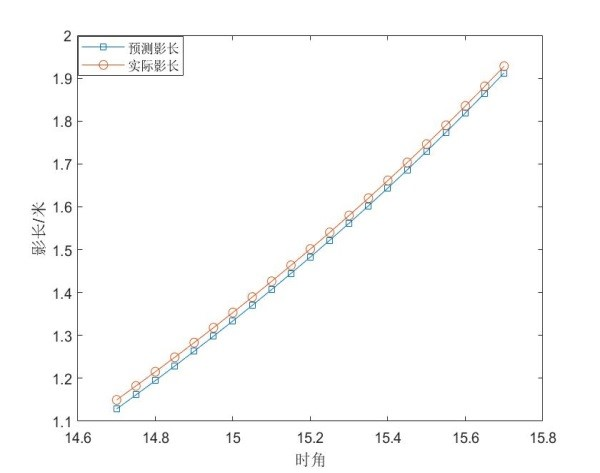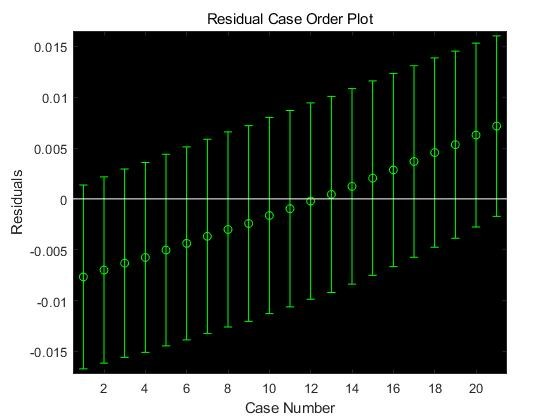clear
clc
%第二问残差检验
L=1.2;%杆长
alpha=119.5;%经度
phi=-10;%纬度
%杆长1.8m时,纬度19N,经度112.5E;杆长1.2m时,纬度-10S,经度119.5E
N=31+28+31+18;%计算该日期下的日数
delta=asind(0.39795*cosd(0.98563*(N-173)));%该日下的赤纬角
A=sind(delta);B=cosd(delta);
t=14.7:0.05:15.7;%设置当天时间范围
omega=15*t+alpha-300;%转化为时角
x=[1.0365,1.0699,1.1038,1.1383,1.1732,1.2087,1.2448,...
1.2815,1.3189,1.3568,1.3955,1.4349,1.4751,1.516,...
1.5577,1.6003,1.6438,1.6882,1.7337,1.7801,1.8277];%杆影x坐标
y=[0.4973,0.5029,0.5085,0.5142,0.5198,0.5255,0.5311,...
0.5368,0.5426,0.5483,0.5541,0.5598,0.5657,0.5715,...
0.5774,0.5833,0.5892,0.5952,0.6013,0.6074,0.6135];%杆影y坐标
d=sqrt(x.^2+y.^2);%当地时角的杆影长
D=L*cotd(asind(A*sind(phi)+B*cosd(phi).*cosd(omega)));%计算的杆影长
figure(1);
plot(D,'s-');hold on;plot(d,'o-');d=d';D=D';
figure(2);
[b,bint,r,rint,stats]=regress(D,d);
rcoplot(r,rint);


### 6.3 模型三： 基与分治思想的循环遍历法——问题三的求解

#### 6.3.1 建模准备

d ′ ( i ) d'(i) 附件2中每个时刻实测影子长度
d ′ ′ ( i ) d''(i) 附件3中每个时刻实测影子长度
d 0 ( i ) d_{0}(i) i i 个时刻实测影子长度 ( i = 1 , 2 , ⋯   , 21 ) (i=1,2,\cdots,21)
d α φ ( i ) d_{\alpha\varphi}(i) 全球范围内任一点( α \alpha 表示该未知的经度坐标, φ \varphi 表示该位置的纬度坐标)第 i i 个时刻的影长

θ α φ ( i ) \theta_{\alpha\varphi}(i) 全球范围内任一点( α \alpha 表示经度, φ \varphi 表示纬度)第个时刻影子方向与第1个时刻影子方向之间的夹角 ( i = 1 , 2 , ⋯   , 20 ) (i=1,2,\cdots,20)
θ i \theta_{i} 附件2中第 i i 个时刻影子方向与 x x 轴的夹角(第 i i 个时刻12:41)
θ i ′ ′ \theta_{i}'' 附件3中第 i i 个时刻影子方向与 x x 轴的夹角(第 i i 个时刻13:09)
θ 0 ( i ) \theta_{0}(i) i i 个时刻影子方向与第1个是时刻影子方向的夹角

θ 0 = ∣ θ 1 − θ i ∣ (6.13) \theta_{0}=|\theta_{1}-\theta_{i}|\tag{6.13} 根据几何知识，有
tan ⁡ θ 1 = y 1 x 1 ， tan ⁡ θ i = y i x i (6.14) \tan\theta_{1}=\frac{y_{1}}{x_{1}}，\tan\theta_{i}=\frac{y_{i}}{x_{i}}\tag{6.14}

#### 6.3.2 多目标优化模型的建立

min ⁡ ∑ i = 1 21 ∣ d α φ ( i ) − d 0 ( i ) ∣ (6.15) \min \sum_{i=1}^{21}|d_{\alpha\varphi}(i)-d_{0}(i)|\tag{6.15} 实际方向角的变化与计算方向角的变化之差之和最小，有
min ⁡ ∑ i = 1 20 ∣ θ α φ ( i ) − θ 0 ( i ) ∣ (6.16) \min \sum_{i=1}^{20}|\theta_{\alpha\varphi}(i)-\theta_{0}(i)|\tag{6.16} 循环搜索的范围，得如下约束条件
s . t . { − 18 0 ∘ ≤ α ≤ 18 0 ∘ − 9 0 ∘ ≤ φ ≤ 9 0 ∘ 0 < L < 6 0 < N < 365 , N 为 整 数 (6.17) \begin{aligned}s.t. \begin{cases} -180^{\circ}\leq\alpha\leq180^{\circ}\\ -90^{\circ}\leq\varphi\leq90^{\circ}\\ 0<L<6\\ 0<N<365,N为整数 \end{cases} \end{aligned}\tag{6.17} 其中， α \alpha 表示经度， φ \varphi 表示纬度， L L 表示直杆的实际长度， N N 表示日期。联立上述(6.15)～(6.17)式，即得循环搜索算法的目标函数与约束条件。

{ d α φ ( i ) = L ⋅ cot ⁡ [ arcsin ⁡ ( sin ⁡ φ sin ⁡ δ + cos ⁡ φ cos ⁡ δ cos ⁡ ω ) ] δ = arcsin ⁡ { 0.39795 ⋅ cos ⁡ [ 0.98563 ⋅ ( N − 173 ) ] } t i = ( t 0 i − 12 ) ⋅ 1 5 ∘ (6.18) \begin{aligned} \begin{cases} d_{\alpha\varphi}(i)=L\cdot \cot[\arcsin(\sin\varphi\sin\delta+\cos\varphi\cos\delta\cos\omega)]\\ \delta=\arcsin\left\{ 0.39795\cdot\cos[0.98563\cdot(N-173)] \right\}\\ t_{i}=(t_{0i}-12)\cdot15^{\circ} \end{cases} \end{aligned}\tag{6.18} 其中݀ d 0 ( i ) = x j 2 + y j 2 d_{0}(i)=\sqrt{x_{j}^{2}+y_{j}^{2}} ，将太阳方位角记为 θ s \theta_{s} ，计算公式为：
cos ⁡ θ s = sin ⁡ ( h ) sin ⁡ φ − sin ⁡ δ cos ⁡ ( h ) ⋅ cos ⁡ φ (6.19) \cos\theta_{s}=\frac{\sin(h)\sin\varphi-\sin\delta}{\cos(h)\cdot\cos\varphi}\tag{6.19} θ α φ ( i ) \theta_{\alpha\varphi}(i) 的计算公式为:
θ α φ ( i ) = ∣ θ s 1 − θ s i ∣ (6.20) \theta_{\alpha\varphi}(i)=|\theta_{s1}-\theta_{si}|\tag{6.20}

#### 6.3.3 模型的求解

##### 3. 模型阶段三：求解结果

11971.907939
21451.907939

1225312769
21453109-27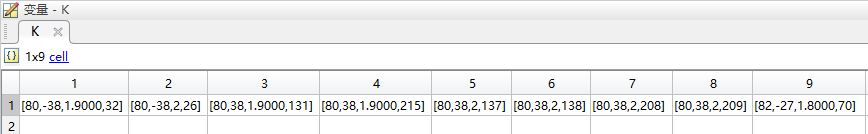clear
clc
%第3问附件2第1次搜索
x=[-1.2352,-1.2081,-1.1813,-1.1546,-1.1281,-1.1018,...
-1.0756,-1.0496,-1.0237,-0.9980,-0.9724,-0.9470,...
-0.9217,-0.8965,-0.8714,-0.8464,-0.8215,-0.7967,...
-0.7719,-0.74730,-0.7227];%杆影的x坐标
y=[0.1730,0.1890,0.2048,0.2203,0.2356,0.2505,...
0.2653,0.2798,0.2940,0.3080,0.3218,0.3354,...
0.3488,0.3619,0.3748,0.3876,0.4001,0.4124,...
0.4246,0.4366,0.4484];%杆影的y坐标
d=sqrt(x.^2+y.^2);%杆影长
K={};k=0;%KK=[];KKK=[];
alpha=0:1:90;%经度
phi=-50:1:50;%纬度
L=1:0.1:3;%杆长
N=1:1:250;%日数
t=12.6833:0.05:13.6833;%拍摄时的北京时间
for i=1:length(alpha)
for j=1:length(phi)
for m=1:length(L)
for n=1:length(N)
flag1=0;flag2=0;%标记
%计算目标函数1影长的误差
delta=asind(0.39795*cosd(0.98563*(N(n)-173)));%赤纬角
A=sind(delta);B=cosd(delta);
omega=15*t+alpha(i)-300;%根据北京时间计算的时角
%计算各地(经纬)不同北京时间的影长
D=L(m)*cotd(asind(A*sind(phi(j))+B*cosd(phi(j)).*cosd(omega)));
%KK1=sum(abs(d-D));KK=[KK,KK1];
if (sum(abs(d-D)))<0.1
flag1=1;%目标函数1满足要求
else
continue
end
%计算目标函数2方向角的误差
theta1=atand(y(1)/x(1));%实测第1个北京时刻与x轴的夹角
theta2=atand(y(2:end)./x(2:end));%实测从第2个北京时刻开始与x轴的夹角
theta0=abs(theta2-theta1);%实测20个时段内与第1个时刻的方位角的变化
%首先求非实测的thetas1
h1=asind(A*sind(phi(j))+B*cosd(phi(j))*cosd(omega(1)));
thetas1=acosd((sind(h1)*sind(phi(j))-sind(delta))/(cosd(h1)*cosd(phi(j))));
h=asind(A*sind(phi(j))+B*cosd(phi(j)).*cosd(omega(2:end)));
%KK2=sum(abs(thetaalpha-theta0));KKK=[KKK,KK2];
if (sum(abs(thetaalpha-theta0)))<0.6
flag2=1;
else
continue
end
if flag1==1 && flag2==1
k=k+1;
list=[alpha(i) phi(j) L(m) N(n)];
K{k}=list;list=[];
end
end
end
end
end
%修改步长进行第二次小范围搜索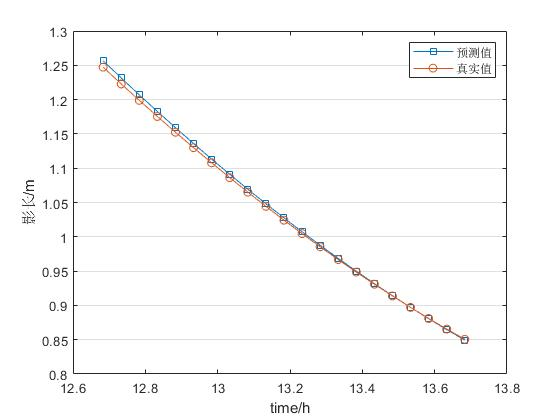clear
clc
%第三问附件2残差检验
L=2;%杆长
alpha=80;%经度
phi=38;%纬度
N=209;%日数
delta=asind(0.39795*cosd(0.98563*(N-173)));%该日下的赤纬角
A=sind(delta);B=cosd(delta);
t=12.6833:0.05:13.6833;%设置当天时间范围
omega=15*t+alpha-300;%转化为时角
x=[-1.2352,-1.2081,-1.1813,-1.1546,-1.1281,-1.1018,...
-1.0756,-1.0496,-1.0237,-0.9980,-0.9724,-0.9470,...
-0.9217,-0.8965,-0.8714,-0.8464,-0.8215,-0.7967,...
-0.7719,-0.74730,-0.7227];%杆影的x坐标
y=[0.1730,0.1890,0.2048,0.2203,0.2356,0.2505,...
0.2653,0.2798,0.2940,0.3080,0.3218,0.3354,...
0.3488,0.3619,0.3748,0.3876,0.4001,0.4124,...
0.4246,0.4366,0.4484];%杆影的y坐标
d=sqrt(x.^2+y.^2);%当地时角的杆影长
D=L*cotd(asind(A*sind(phi)+B*cosd(phi).*cosd(omega)));%计算的杆影长
figure(1);
plot(t,D,'s-');hold on;plot(t,d,'o-');d=d';D=D';set(gca,'ygrid','on');
legend("预测值","真实值");xlabel("time/h");ylabel("影长/m");
figure(2);
[b,bint,r,rint,stats]=regress(D,d);
rcoplot(r,rint);


### 6.4 模型四：基与坐标变换的图形射影模型——问题四的求解

#### 6.4.1 建模准备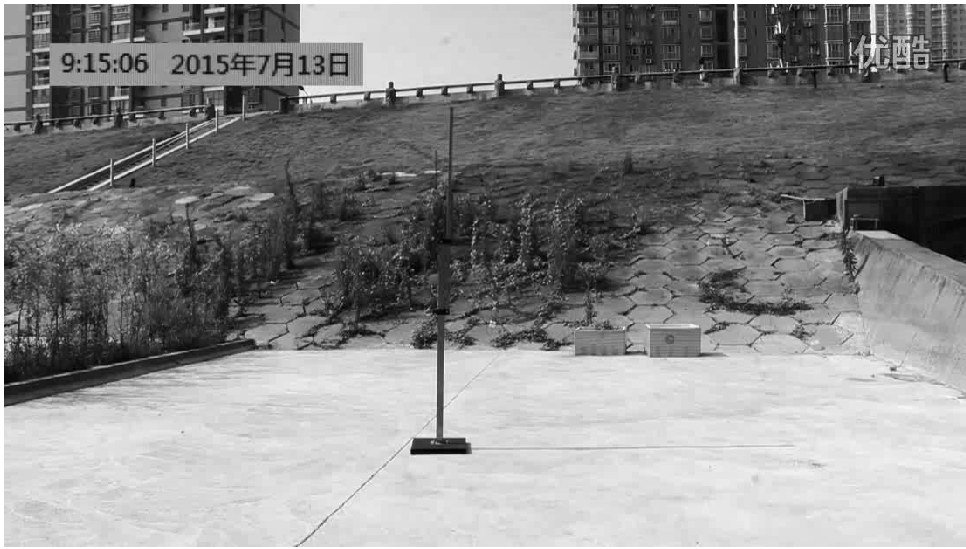## 附录

09-09
04-2608-20
03-152720
03-18372
09-118328
12-041860
09-0743
07-26186
05-25178
06-12553
04-197935
10-272033
01-291万+
06-03105点击重新获取扫码支付余额充值